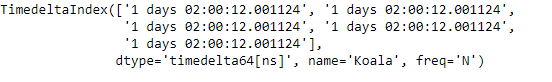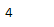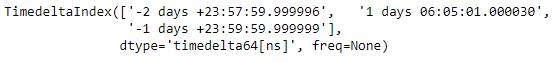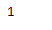Related Articles
Python | Pandas TimedeltaIndex.argmax
• Last Updated : 28 Oct, 2019

Python is a great language for doing data analysis, primarily because of the fantastic ecosystem of data-centric python packages. Pandas is one of those packages and makes importing and analyzing data much easier.

Pandas` TimedeltaIndex.argmax()` function returns the indices of the maximum values along an axis in the TimedeltaIndex object. The function is similar to ` numpy.ndarray.argmax`.

Syntax : TimedeltaIndex.argmax(axis=None, *args, **kwargs)

Parameters :
axis : None

Return : integer index value

Example #1: Use `TimedeltaIndex.argmax()` function to find the indices of the maximum value in the given TimedeltaIndex object.

 `# importing pandas as pd ` `import` `pandas as pd ` ` `  `# Create the first TimedeltaIndex object ` `tidx ``=` `pd.TimedeltaIndex(start ``=``'1 days 02:00:12.001124'``,  ` `                   ``periods ``=` `5``, freq ``=``'N'``, name ``=``'Koala'``) ` ` `  `# Print the TimedeltaIndex object ` `print``(tidx) `

Output :Now we will print the index of the maximum value in tidx object.

 `# return the index of maximum value. ` `tidx.argmax() `

Output :As we can see in the output, the `TimedeltaIndex.argmax()` function has 4 indicating that the maximum value in tidx object is present at 4th index.

Example #2: Use `TimedeltaIndex.argmax()` function to find the indices of the maximum value in the given TimedeltaIndex object.

 `# importing pandas as pd ` `import` `pandas as pd ` ` `  `# Create the TimedeltaIndex object ` `tidx ``=` `pd.TimedeltaIndex(data ``=``[``'-1 days 2 min 3us 10ns'``,  ` `                                ``'1 days 06:05:01.000030'``, ` `                                ``'-1 days + 23:59:59.999999'``]) ` ` `  `# Print the TimedeltaIndex object ` `print``(tidx) `

Output :Now we will print the index of the maximum value in tidx object.

 `# return the index of maximum value. ` `tidx.argmax() `

Output :As we can see in the output, the `TimedeltaIndex.argmax()` function has 1 indicating that the maximum value in tidx object is present at 1st index.

Attention geek! Strengthen your foundations with the Python Programming Foundation Course and learn the basics.

To begin with, your interview preparations Enhance your Data Structures concepts with the Python DS Course.

My Personal Notes arrow_drop_up
Recommended Articles
Page :